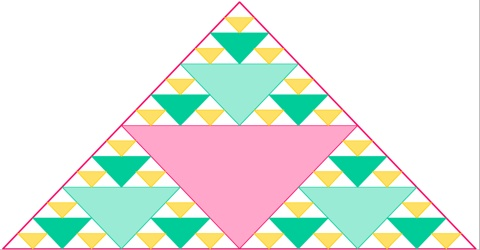Mathematic

# Triangles: Definition with TypesTriangle is a plane figure with three straight sides and three angles. The three angles always add to 180°. The vertex is a corner of the triangle. Every triangle has three vertices. The interior angles of a triangle always add up to 180°. The exterior angles of a triangle always add up to 360°.Types of triangles

Types of triangles may be classified by their sides, by their angles or by a combination of both sides and angles.

Triangles classified by their sides:

• Scalene triangle: No equal sides and no equal angles. A scalene triangle is a triangle that has no equal sides.
• Isosceles triangle: Two equal sides and two equal angles. An isosceles triangle is a triangle that has two equal sides.
• Equilateral triangle: Three equal sides and three equal angles, always 60°. An equilateral triangle is a triangle that has three equal sides.Triangles classified by their angles:

• Right triangle: A right triangle has a 90 degrees angle.
• Obtuse triangle: An obtuse triangle has one angle that is bigger than 90 degrees (Obtuse angle).
• Acute triangle: In an acute triangle, all angle are less than 90 degrees, so all angles are acute angles.